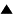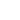# Verification

[Node to be completed]

Verification is a direct use of the definition of truth to decide if the statement is true.

Predictions are, in principle, verifiable. You only have to initiate the test process that the prediction is about and wait until it comes to the final state. Thus, if a prediction is correct, there is, in principle, a way to verify this, even though it may require such a huge time, that in practice it is impossible.

Now consider the situation when you want to verify a prediction T!, but the test process T is infinite. This means that the prediction is false, but you will never be able to verify this directly. To sum up, the following three cases are possible when we try to verify a prediction:

```       Testing process                  Prediction is
--------------------            ------------------
1.    ends in success                   verifyably true
2.    ends in failure                   verifyably false
3.       never ends             false, but this is not directly verifyable
```

As to the verififcation of general statements (generators of predictions), it may be possible only if the number of prediction produced is finite. In the more usual case, with an infinite number of prediction produced, the direct verification is impossible.

 HomeMetasystem Transition TheoryEpistemologyKnowledgeTruthProof Up Prev.Next Down